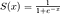# An Introduction into gRPC

This is a basic introduction of gRPC.

# Benefits of gRPC

gRPC uses protocol buffers and HTTP2, which will help the service communications to be much faster, and use less resources.

## Protocol Buffers vs JSON

By using protocol buffers, we can achieve faster and more efficient communication, especially for devices with slower CPUs:

# Zero to Hero — Master Kafka Tutorial 1 (Basic Theory)

This is the first tutorial of this series, which covers the basic concepts of Kafka

# Google Interview Question— LeetCode 1055

In this blog, we will discuss an interview question asked by Google.

In this post, we are going to discuss the leetcode problem no. 1055 — Shortest Way to Form String, which is recently asked in Google interviews.

Firstly, we will try to solve the problem with brute force method in O(n²) running time; then we will improve our algorithm to O(n log n) running time; finally we will further improve the algorithm to O(n).

# Problem Analysis

The input of the problem is two strings: `source` and `target`. …

# Ace the Coding Interview — LeetCode 253

Recent interview question asked by Google and Amazon.

In this post, we are going to discuss the leetcode problem no. 253 — Meeting Rooms II. This problem is recently asked in Google and Amazon interviews.

# Problem Analysis

The input of the problem is a list of meeting schedules. Each meeting schedule is represented by a list of two items, with the first item as the starting time of a meeting, and the second item as the ending time of the meeting.

The expected output of the problem is an integer, which is the minimum number of meeting rooms required to fulfill…

# Data Structure and Algorithms — Implement Dynamic Array in Python

This is an introduction to dynamic array and its implementation

An array is a contiguous area of memory of equal-size elements. Array length is usually fixed, which means you need to specify the number of elements your array can hold ahead of time.

A dynamic array is an array which has an important feature: auto-resizing. With this feature, you can easily expand your array by adding more elements in it, so that you do not need to determine the size ahead of time.

In this tutorial, we will discuss about the implementation of dynamic array by using regular fixed length…

# Understand the Math for Neural Networks

## Detailed explanation for Gradient Descent and Back-propagation in math

In this post, I will discuss details about Gradient Descent and Back-propagation in neural networks, and will help you to understand why there is Vanishing Gradient Problem. In my earlier post (How to implement Gradient Descent in Python), I discussed python implementation of Gradient Descent. In this post, I will show you step-by-step to understand the math behind the Neural Networks.

# Assumptions

For the Neural Networks discussed in the post, we will make the following assumptions:Sigmoid Function

# How to implement Gradient Descent in Python

## This is a tutorial to implement Gradient Descent Algorithm for a single neuron

Gradient Descent is the most important concept in Neural Networks. In this tutorial, I am going to show you how to implement it in Python. I hope this tutorial can help you to build a better understanding about how gradient descent works, and how it helps to improve model accuracy.

# Data Pre-Processing

We will try to build a single neuron network, which can predict the admissions of a graduate school. The data we will use is shared above in google drive. Let us first take a peek at the raw data:

The first 5 rows of data are shown below. The…## Y Tech

Software Engineer